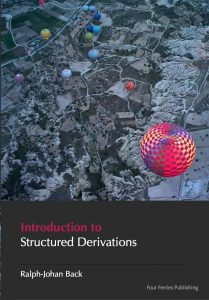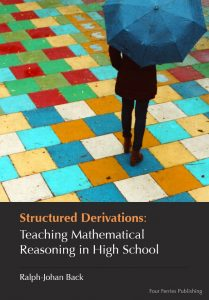# Teaching Methodology# Tutorials

### Learn to teach with Structured Derivations

Learn how to teach using Structured Derivations. The tutorial will introduce you to how to structure the calculations, how to introduce the calculations environment with facts, assumptions and declarations , and how to make calculations with sub-derivations.

### Tutorial on Structured Derivation

Find out more about structured derivations.  The tutorials are in PDF format, and are freely downloadable from the web site.Structured Derivations: Teaching Mathematical Reasoning in High school

This book is intended for mathematics teachers who want to try out the method  in practice in teaching. It shows how to structure a mathematical derivation so that it is easy to see the overall structure of the argument. Book is available at Amazon.### Textbooks on Structured Derivations

Four Ferries Publishing offers two textbooks on structured derivations. The first one gives an overall description of the method, and explains by a large number of examples how to use the method in practical education at high school level. The second book is a handbook on structured derivations,  covering both the method and its logical foundation. Both books are  intended for mathematics teachers on all levels of math education, to help them make their explanations easier to understand and follow.

Teaching Mathematics in the Digital Age with Structured Derivations

This book extends the first book with a more thorough treatment of the logic behind high school mathematics, as well as the logical basis of the method. It also goes further, presenting material that is directed for introductory level university mathematics. The book is available at Amazon